Daily Practice Problems
Class 7 Maths
Lines and AnglesQuestion 1:

The angles between North and West and South and East are:

(a) complementary                        (b) Supplementary

(c) both are acute                          (d) both are obtuse

Question 2:

Angles between South and West and South and East are

(a) Vertically opposite angles

(b) complementary angles

(c) Making a linear pair

Question 3:

In the given fig., PQ is a mirror, AB is the incident ray and BC is the reflected ray. If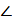ABC = 460. FindABP is equal to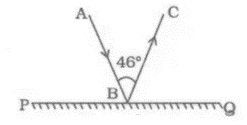Question 4:

In the figure, lines PQ and ST intersect at O. If POR = 90° and x : y = 3 : 2, then find the value of z.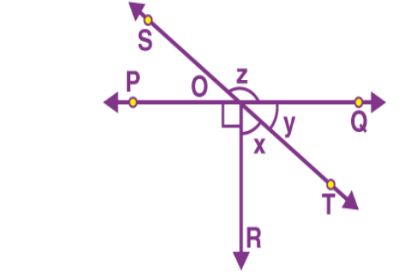Question 5:

3a + 16 and 2a + 34 are supplementary angles. Find a.

Question 6:

In the figure, PQ||ST. Find the value of x + 2y.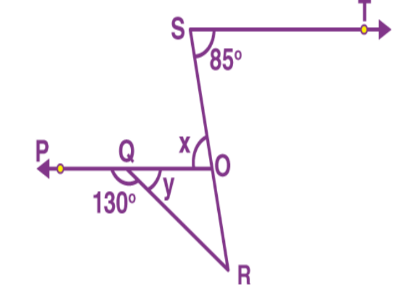Question 7:

In the given figure, find the value of x.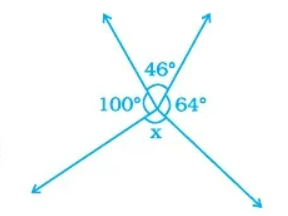Question 8:

In the given fig., if AB ||CDAPQ = 500 andPRD =1300, findQPR.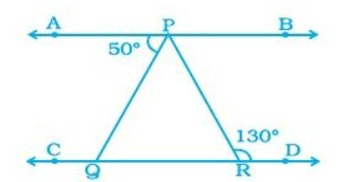Question 9:

In the given fig., lines l and m intersect each other at a point. Which of the following is false.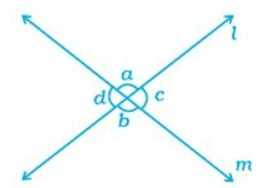(a)   a =  b                                     (b)  d =  c

(c)  a +  d = 1800                         (d)  a =  d

Question 10:

If the complement of an angle is 620, find its supplement.

Question 11:

In the given figure, two parallel line l and m are cut by two transversal n and p. Find the values of x and y.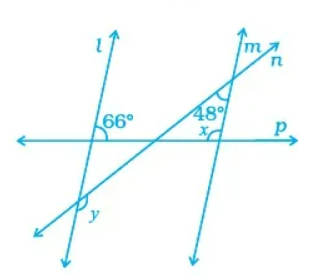Question 12:

Find the value of x.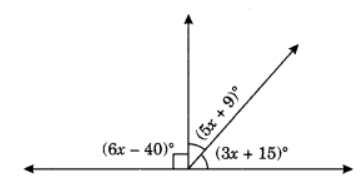Question 13:

In the given figure, three lines AB, CD and EF intersect each other at O. IfAOE = 300 andDOB = 400 FindCOF.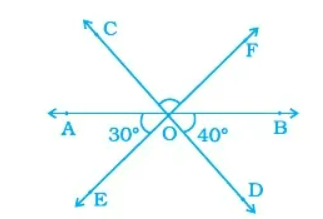Question 14:

In the given figure, OB is perpendicular to OA andBOC = 490. FindAOD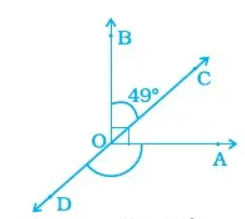Question 15:

State ‘True’ or ‘False’:

(1) Two right angles are complementary to each other.

(2) One obtuse angle and one acute angle can make a pair of complementary angles.

(3) Two supplementary angles are always obtuse angles.

Question 16:

Fill in the blanks:

(1) Two angles forming a _____ pair are supplementary.

(2) Two lines in a plane which do not meet at a point anywhere are called _____ lines.

(3) Sum of interior angles at the same side of transversal is _____.

Question 17:

What is the type of other angle of a linear pair if

(a) one of its angles is acute?

(b) one of its angles is obtuse?

(c) one of its angles is right?

Question 18:

In the given figure, PQ || ST, find the value of x + y.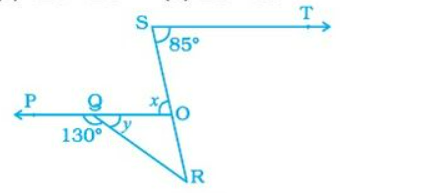Question 19: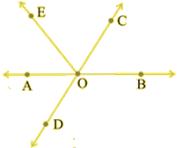In the given figure, identify:

(i) Five pairs of adjacent angles.

(ii) Three linear pairs.

(iii) Two pairs of vertically opposite angles.

Question 20: